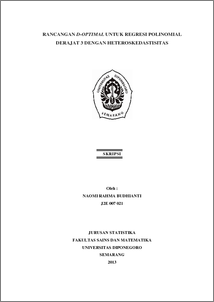# RANCANGAN D-OPTIMAL UNTUK REGRESI POLINOMIAL DERAJAT 3 DENGAN HETEROSKEDASTISITAS

BUDHIANTI, NAOMI RAHMA (2013) RANCANGAN D-OPTIMAL UNTUK REGRESI POLINOMIAL DERAJAT 3 DENGAN HETEROSKEDASTISITAS. Undergraduate thesis, UNIVERSITAS DIPONEGORO.Preview
PDF
480Kb

## Abstract

An experiment, the 3rd degree of polynomial regression model with heteroscedastic and containing function of weight λ(x)=x e^(-x) uses D-Optimal criteria. The D-Optimal criteria is obtained by maximizing determinant of design matrix or minimizing determinant of design matrix inverse. The design matrix is formed from optimal design. The optimal design is a matrix that consists the points and proportions at each point. The points are generated by the Laguerre polynomial roots. The D-Optimal design that has maximum value of standardized variance equals to amount of parameters at every point qualifies as a D-Optimal local design. Keywords : Polynomial Regression, Heteroscedastic, D-Optimal Design, Laguerre Polynomial

Item Type: Thesis (Undergraduate) H Social Sciences > HA Statistics Faculty of Science and Mathematics > Department of Statistics 42336 Mr Hasbi Yasin 20 Feb 2014 09:19 04 Mar 2014 11:11

Repository Staff Only: item control page